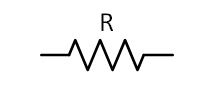# Some resistor questions and their true answersHi. Hope you are having a good time. In this post, I cover some really good resistor questions and try to answer them with my knowledge best. These questions include some basic as well as advanced level questions. Hopefully, you will find this post helpful.

## Basic Resistor Questions and their Answers

I will start with some basic resistor questions and then will go towards some advanced one. I cannot say for sure how many questions I will answer but I will do my best and try to update this post as I learn new thing about the resistor.

## What is a resistor?

A resistor is two terminal linear electronic device which is used to restrict electric current flow in a circuit. It is linear because it obeys Ohm’s law.

## Is resistor an active or passive device?

The resistor is a passive device as it dissipates energy in the form of heat.

## What is the electrical symbol of a resistor?## Why is the symbol of the resistor zig zag?

This question has no technical answer, it is truly a subjective type of question. I answer it like, a resistor resists current low making it hard for current to flow through it. To show it graphically zig-zag path is the best, as the zig-zag path represents a hard path to pass by it.

## Who invented the resistor?

Otis Frank Boykin invented the resistor, born on August 29, 1920, in Dalla.

## Why resistors have no polarity?

Resistors have no polarity, they are non-polar because of its design. They are designed in a way that you can connect it in either direction in a circuit its function remains the same.

## What are resistors made of?

Most common, modern resistors are made out of either a carbon, metal or metal oxide film.

## What resistor tolerance mean?

Tolerance is the percentage of error in the resistor’s resistance, or how much more or less you can expect a resistor’s actual measured resistance to be from its stated resistance. A gold tolerance band is 5% tolerance, silver is 10%, and no band at all would mean a 20% tolerance. (Parallax)

## What does the s color code of resistor do?

The color code is used to tell the resistance value of a resistor without using a multimeter. You can say they are the color bars printed on the body of the resistor showing the actual value of the resistor.

## In which unit resistance of the resistor is measured?

The measuring unit of resistance of a resistor is Ohm. There are so many standard values of resistance i.e. in kOhms, MOhms etc.

Now, let see some advanced resistor questions and try to answer each of them. If you have any question not answer in the post you can ask it in the comments section. Feel free to ask any question. I really do appreciate it.

## Why does the resistor get heated?

The electric current flow through the resistor constantly, when the circuit is powered up. We know current flow in the form of free electrons. These free-moving electrons cause heat due to the fraction phenomenon inside the resistor body. And this fraction caused the resistor to get heated. This heat energy can be calculated by the following equation:$Heat \, energy= I^{2}R$

## How to measure the value of resistance?

The value of resistance of a resistor can find out by two methods, by color code, or by using a multimeter.

## Why resistor burn out?

A resistor has some power rating which you can find out in its datasheet. Once you exceed the power limits you end in burning the resistor. The power rating exceeds in two ways, either you increase the current through the resistor or you increase the voltage across it.

Other useful posts

Scroll to Top